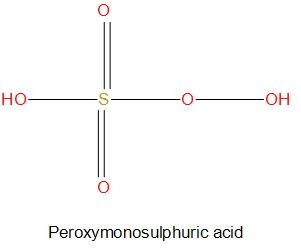QuestionAnswers

# The oxidation numbers of the sulphur atoms in peroxomonosulphuric acid ${{H}_{2}}S{{O}_{5}}$and peroxodisulphuric acid ${{H}_{2}}{{S}_{2}}{{O}_{8}}$are respectively.A. +8and +7B. +3 and +3C. +6 and+6D. +4 and +6Verified
128.7k+ views
Hint:
To find the oxidation numbers we should know the charges present on each atom. Here, the compounds given are having peroxy linkage that is there is presence of a O-O single bond.

- Let’s first find the oxidation state of sulphur in ${{H}_{2}}S{{O}_{5}}$,
If we find oxidation state, consider sulphur as x, then it will be:
\begin{align} & 2\times \left( 1 \right)+x+5\times \left( -2 \right)=0 \\ & 2+x+\left( -10 \right)=0 \\ & x=+8 \\ \end{align}
We get the oxidation state as +8, but this is not true, because the maximum oxidation number for sulphur cannot exceed +6. Since sulphur has only 6 electrons in its valence shell.
-We get this exceptional value of oxidation state for sulphur in${{H}_{2}}S{{O}_{5}}$ due to the peroxy linkage shown by two atoms.- We can see from the structure of ${{H}_{2}}S{{O}_{5}}$, there are two peroxide molecules present and all the other oxygen atoms are normally attached. Here we will consider the charge on three oxygen atoms as -2 and charge on two peroxide molecules as -1. So, if we find the oxidation state of sulphur, then we get:
\begin{align} & 2\times \left( 1 \right)+2\times \left( -1 \right)+3\times \left( -2 \right)+x=0 \\ & 2+\left( -2 \right)+\left( -6 \right)+x=0 \\ & x=+6 \\ \end{align}
Hence, the oxidation state of ${{H}_{2}}S{{O}_{5}}$is +6.
- Let’s next find the oxidation state of sulphur in${{H}_{2}}{{S}_{2}}{{O}_{8}}$:
If we find oxidation state, consider sulphur as x, then it will be:
\begin{align} & 2\times \left( 1 \right)+2\times x+8\times \left( -2 \right)=0 \\ & 2+2x+\left( -16 \right)=0 \\ & x=+7 \\ \end{align} 
We get the oxidation state as +7, but this is not true, because the maximum oxidation number for sulphur cannot exceed +6. Since sulphur has only 6 electrons in its valence shell.
-We get this exceptional value of oxidation state for sulphur in ${{H}_{2}}{{S}_{2}}{{O}_{8}}$ due to the peroxy linkage shown by two atoms.- We can see from the structure of ${{H}_{2}}{{S}_{2}}{{O}_{8}}$, there are two peroxide molecules present and all the other oxygen atoms are normally attached. Here we will consider the charge on six oxygen atoms as -2 and charge on two peroxide molecules as -1. So, if we find the oxidation state of sulphur, then we get:
\begin{align} & 2\times \left( 1 \right)+2\times x+2\times \left( -1 \right)+6\times \left( -1 \right)=0 \\ & 2+2x+\left( -2 \right)+\left( -12 \right)=0 \\ & 2+2x-2-12=0 \\ & 2x=12 \\ & x=+6 \\ \end{align}
Hence, the oxidation state of ${{H}_{2}}{{S}_{2}}{{O}_{8}}$ is +6.

Hence, the correct option is C.

That is the oxidation numbers of the sulphur atoms in peroxomonosulphuric acid ${{H}_{2}}S{{O}_{5}}$ and peroxodisulfuric acid ${{H}_{2}}{{S}_{2}}{{O}_{8}}$ are respectively +6 and+6.

Note:
- It is to be noted here that there is peroxy linkage shown by two oxygen atoms, and therefore in such cases we have to consider the separate charge of two peroxide molecules and then should find the oxidation state of the central metal atoms.